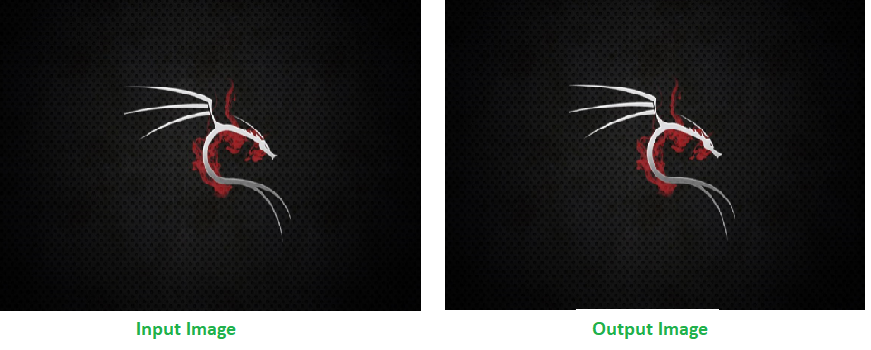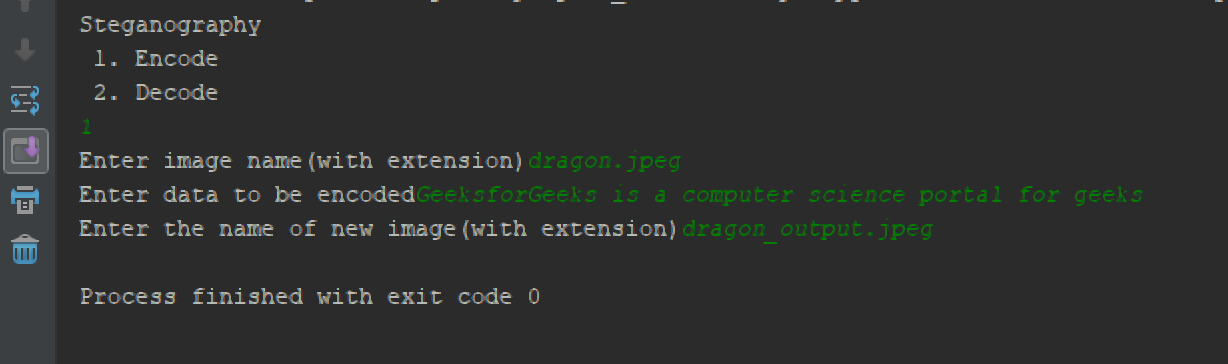# Image based Steganography using Python

Steganography is the method of hiding secret data in any image/audio/video. In a nutshell, the main motive of steganography is to hide the intended information within any image/audio/video that doesn’t appear to be secret just by looking at it.
The idea behind image-based Steganography is very simple. Images are composed of digital data (pixels), which describes what’s inside the picture, usually the colors of all the pixels. Since we know every image is made up of pixels and every pixel contains 3-values (red, green, blue).

### Encode the data :

Every byte of data is converted to its 8-bit binary code using ASCII values. Now pixels are read from left to right in a group of 3 containing a total of 9 values. The first 8-values are used to store binary data. The value is made odd if 1 occurs and even if 0 occurs.
For example :
Suppose the message to be hidden is ‘ Hii ‘. Since the message is of 3-bytes, therefore, pixels required to encode the data is 3 x 3 = 9. Consider a 4 x 3 image with a total 12-pixels, which are sufficient to encode the given data.

```[(27, 64, 164), (248, 244, 194), (174, 246, 250), (149, 95, 232),
(188, 156, 169), (71, 167, 127), (132, 173, 97), (113, 69, 206),
(255, 29, 213), (53, 153, 220), (246, 225, 229), (142, 82, 175)]

```

ASCII value of ‘ H ‘ is 72 whose binary equivalent is 01001000.
Taking first 3-pixels (27, 64, 164), (248, 244, 194), (174, 246, 250) to encode. Now change the pixel to odd for 1 and even for 0. So, the modifies pixels are (26, 63, 164), (248, 243, 194), (174, 246, 250). Since we have to encode more data, therefore, the last value should be even. Similarly, ‘i‘ can be encoded in this image.
The new image will look like :

```[(26, 63, 164), (248, 243, 194), (174, 246, 250), (148, 95, 231),
(188, 155, 168), (70, 167, 126), (132, 173, 97), (112, 69, 206),
(254, 29, 213), (53, 153, 220), (246, 225, 229), (142, 82, 175)]

```

### Decode the data :

To decode, three pixels are read at a time, till the last value is odd, which means the message is over. Every 3-pixels contain a binary data, which can be extracted by the same encoding logic. If the value if odd the binary bit is 1 else 0.Below is the implementation of the above idea :

## Python

 `# Python program implementing Image Steganography`   `# PIL module is used to extract` `# pixels of image and modify it` `from` `PIL ``import` `Image`   `# Convert encoding data into 8-bit binary` `# form using ASCII value of characters` `def` `genData(data):`   `        ``# list of binary codes` `        ``# of given data` `        ``newd ``=` `[]`   `        ``for` `i ``in` `data:` `            ``newd.append(``format``(``ord``(i), ``'08b'``))` `        ``return` `newd`   `# Pixels are modified according to the` `# 8-bit binary data and finally returned` `def` `modPix(pix, data):`   `    ``datalist ``=` `genData(data)` `    ``lendata ``=` `len``(datalist)` `    ``imdata ``=` `iter``(pix)`   `    ``for` `i ``in` `range``(lendata):`   `        ``# Extracting 3 pixels at a time` `        ``pix ``=` `[value ``for` `value ``in` `imdata.__next__()[:``3``] ``+` `                                ``imdata.__next__()[:``3``] ``+` `                                ``imdata.__next__()[:``3``]]`   `        ``# Pixel value should be made` `        ``# odd for 1 and even for 0` `        ``for` `j ``in` `range``(``0``, ``8``):` `            ``if` `(datalist[i][j] ``=``=` `'0'` `and` `pix[j]``%` `2` `!``=` `0``):` `                ``pix[j] ``-``=` `1`   `            ``elif` `(datalist[i][j] ``=``=` `'1'` `and` `pix[j] ``%` `2` `=``=` `0``):` `                ``if``(pix[j] !``=` `0``):` `                    ``pix[j] ``-``=` `1` `                ``else``:` `                    ``pix[j] ``+``=` `1` `                ``# pix[j] -= 1`   `        ``# Eighth pixel of every set tells` `        ``# whether to stop ot read further.` `        ``# 0 means keep reading; 1 means thec` `        ``# message is over.` `        ``if` `(i ``=``=` `lendata ``-` `1``):` `            ``if` `(pix[``-``1``] ``%` `2` `=``=` `0``):` `                ``if``(pix[``-``1``] !``=` `0``):` `                    ``pix[``-``1``] ``-``=` `1` `                ``else``:` `                    ``pix[``-``1``] ``+``=` `1`   `        ``else``:` `            ``if` `(pix[``-``1``] ``%` `2` `!``=` `0``):` `                ``pix[``-``1``] ``-``=` `1`   `        ``pix ``=` `tuple``(pix)` `        ``yield` `pix[``0``:``3``]` `        ``yield` `pix[``3``:``6``]` `        ``yield` `pix[``6``:``9``]`   `def` `encode_enc(newimg, data):` `    ``w ``=` `newimg.size[``0``]` `    ``(x, y) ``=` `(``0``, ``0``)`   `    ``for` `pixel ``in` `modPix(newimg.getdata(), data):`   `        ``# Putting modified pixels in the new image` `        ``newimg.putpixel((x, y), pixel)` `        ``if` `(x ``=``=` `w ``-` `1``):` `            ``x ``=` `0` `            ``y ``+``=` `1` `        ``else``:` `            ``x ``+``=` `1`   `# Encode data into image` `def` `encode():` `    ``img ``=` `input``(``"Enter image name(with extension) : "``)` `    ``image ``=` `Image.``open``(img, ``'r'``)`   `    ``data ``=` `input``(``"Enter data to be encoded : "``)` `    ``if` `(``len``(data) ``=``=` `0``):` `        ``raise` `ValueError(``'Data is empty'``)`   `    ``newimg ``=` `image.copy()` `    ``encode_enc(newimg, data)`   `    ``new_img_name ``=` `input``(``"Enter the name of new image(with extension) : "``)` `    ``newimg.save(new_img_name, ``str``(new_img_name.split(``"."``)[``1``].upper()))`   `# Decode the data in the image` `def` `decode():` `    ``img ``=` `input``(``"Enter image name(with extension) : "``)` `    ``image ``=` `Image.``open``(img, ``'r'``)`   `    ``data ``=` `''` `    ``imgdata ``=` `iter``(image.getdata())`   `    ``while` `(``True``):` `        ``pixels ``=` `[value ``for` `value ``in` `imgdata.__next__()[:``3``] ``+` `                                ``imgdata.__next__()[:``3``] ``+` `                                ``imgdata.__next__()[:``3``]]`   `        ``# string of binary data` `        ``binstr ``=` `''`   `        ``for` `i ``in` `pixels[:``8``]:` `            ``if` `(i ``%` `2` `=``=` `0``):` `                ``binstr ``+``=` `'0'` `            ``else``:` `                ``binstr ``+``=` `'1'`   `        ``data ``+``=` `chr``(``int``(binstr, ``2``))` `        ``if` `(pixels[``-``1``] ``%` `2` `!``=` `0``):` `            ``return` `data`   `# Main Function` `def` `main():` `    ``a ``=` `int``(``input``(``":: Welcome to Steganography ::\n"` `                        ``"1. Encode\n2. Decode\n"``))` `    ``if` `(a ``=``=` `1``):` `        ``encode()`   `    ``elif` `(a ``=``=` `2``):` `        ``print``(``"Decoded Word :  "` `+` `decode())` `    ``else``:` `        ``raise` `Exception(``"Enter correct input"``)`   `# Driver Code` `if` `__name__ ``=``=` `'__main__'` `:`   `    ``# Calling main function` `    ``main()`

Output :Attention geek! Strengthen your foundations with the Python Programming Foundation Course and learn the basics.

To begin with, your interview preparations Enhance your Data Structures concepts with the Python DS Course.

My Personal Notes arrow_drop_upAppsecco | Web developer | IEEE | GeeksforGeeks Intern | Aspiring Cyber Security Specialist | Camcann Visit https//ashwingoelcom

If you like GeeksforGeeks and would like to contribute, you can also write an article using contribute.geeksforgeeks.org or mail your article to contribute@geeksforgeeks.org. See your article appearing on the GeeksforGeeks main page and help other Geeks.

Please Improve this article if you find anything incorrect by clicking on the "Improve Article" button below.

Article Tags :
Practice Tags :

5

Please write to us at contribute@geeksforgeeks.org to report any issue with the above content.